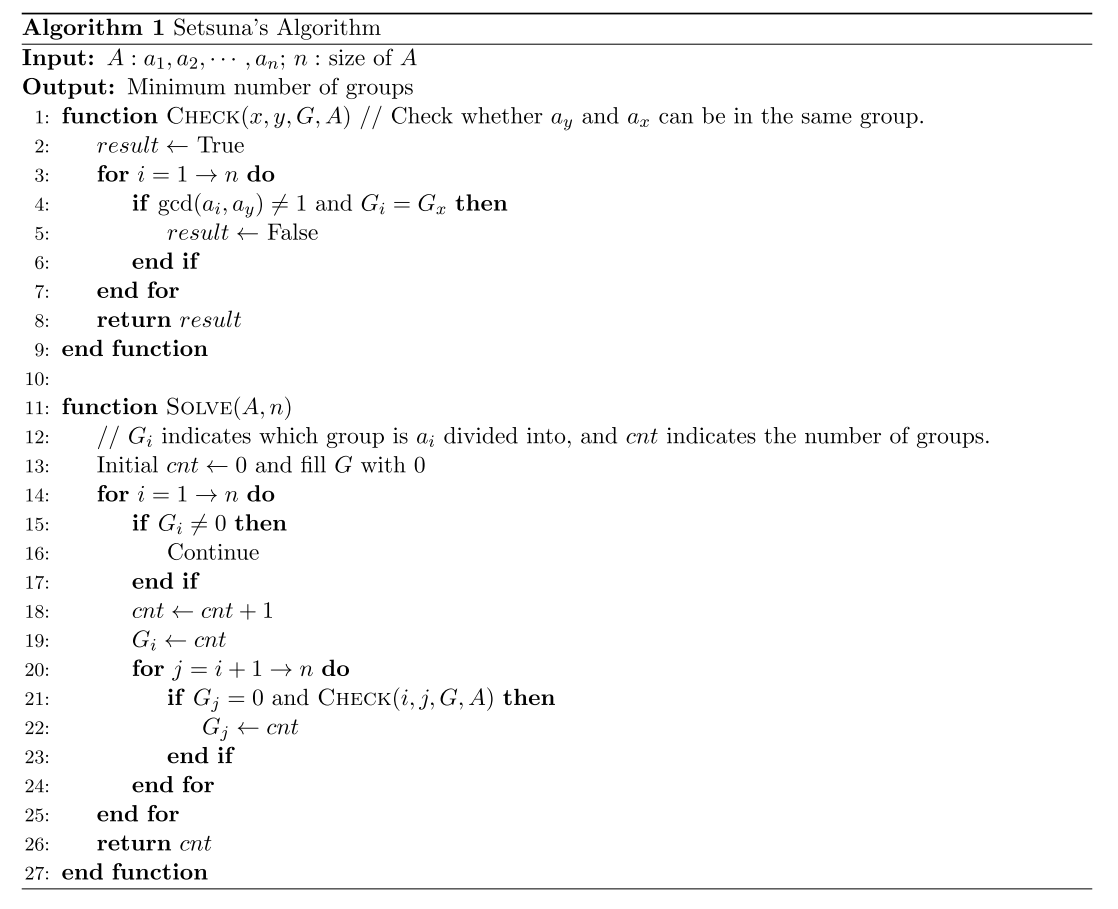# 2020 年 “联想杯”全国高校程序设计在线邀请赛暨第三届上海理工大学程序设计竞赛

F. Fake Algorithm
PDF 题面可用

Setsuna has been fascinated by coprime pairs recently, so she decided to play a game about numbers.

Here are $n$ integers $a_1,a_2,\cdots,a_n(2 \leq a_i \leq 10^{18})$. Setsuna wants to divide the numbers into as few groups as possible so that each number is exactly in one group and the numbers in each group are coprime with each other.

We say that positive integers $x$ and $y$ are coprime if and only if $\gcd(x,y)=1$, where $\gcd(x,y)$ refers to their greatest common divisor.

However, Setsuna is not good at math, so she only comes up with a fake algorithm.Her greedy algorithm is obviously wrong, so we need to hack her solution.

You are given one integer $k$. You need to find the input consisting of $n(n \leq 300)$ integers and a corresponding partition to the input(not necessarily be the minimum partition), which satisfies $X=Y+k$, where $Y$ is the number of groups of your partition and $X$ is the answer of her algorithm to your input.

It can be proved that the answer always exists.

### 输入格式

The input contains one integer $k(1 \leq k \leq 7)$.

### 输出格式

In the first line, output two integers $n,Y(1 \leq Y \leq n \leq 300)$.

In the second line, output $n$ integers $a_1,a_2,\cdots,a_n(2 \leq a_i \leq 10^{18})$, separated by spaces.

In the third line, output $n$ integers $G_1,G_2,\cdots,G_n(1 \leq G_i \leq Y)$, separated by spaces, where $g_i$ indicates which group is $a_i$ divided into.

If there are multiple solutions, you can output any.

### 样例

Input
1

Output
4 2
8 3 45 100
1 2 1 2


### 提示

In the sample, Setsuna will get $3$ groups: $\{8,3\},\{45\},\{100\}$, but we have a better partition: $\{8,45\},\{3,100\}$.

NaN